Algebra problems 21 to 29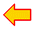problems 11 to 20

21.
Given are:
A+B+C = 81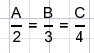Calculate B.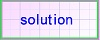22.
Given is:
x2y2 + x2 + y2 - 6x - 6y + 10 = 0.

Calculate x2+y2.23.
A and B are constants.
If
x2 + xy = A .....1)
xy + y2 = B .....2)

calculate the values of x and y.24.
Solve x in the equation:25.
If:
a + b + c = 1 and
a3 + b3 + c3 = 4
calculate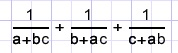26.
A right angled triangle has an area of 30 (square meter) and a perimeter of 30 (meter).

Calculate the sides.27.
Calculate using pencil and paper: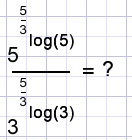28.
Prove that n3+5n is a multiple of 6. {n=1,2,3....}29.
solve x if

a).....x3log(x)=3
b).....x3log(2x)=6